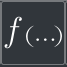# Scalars

The Scalars category consists of 5 nodes that relate to the use of scalars and mathematical expressions as inputs and outputs.Scalar Input

Use a scalar quantity (any integer or decimal) as an inputScalar Output

Display a scalar outputScalar Expression

Combine or modify scalars with an arithmetic operationGet Real Part

Get the real part of a complex numberGet Imaginary Part

Get the imaginary part of a complex number